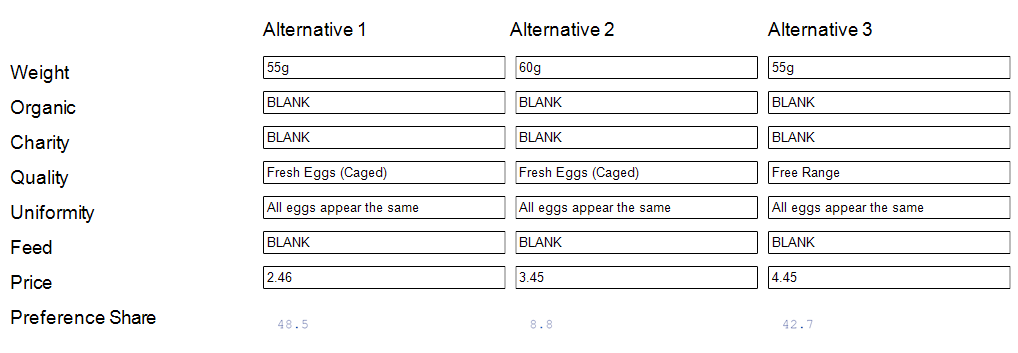# Choice Modeling - Simulator

Create a conjoint/choice model simulator

Create a simulator for a choice model in Displayr. This feature is not available in Q.

## How to Create

To create a simulator, in the object inspector of a choice model output from latent class analysis, hierarchical Bayes, or multinomial logit, click the Simulator extension button. This extension button can be found under Inputs > SIMULATION > Simulator.

A simulator can also be created by first selecting your choice model output and then selecting Anything > Advanced Analysis > Choice Modeling > Simulator from the ribbon.

You will be prompted to enter the number of alternatives you would like in the simulator. If your choice model was fit using an Alternative attribute (i.e. alternative-specific constants), you will be asked whether you want to include them as an attribute in the simulator. Finally, if your choice model includes a 'None' alternative, you will be asked if you would like to include that as well. A new page will be created in your document immediately after your choice model containing the simulator.

## Example

Example Input:
The following choice model was fit using hierarchical Bayes to a conjoint experiment examining preferences for purchasing eggs in a supermarket.

Output Example:
The resulting simulator after requesting 3 alternatives and choosing to not include the Alternative attribute is as follows. (Note, some Combo Boxes have been changed from their default values.)## Options

Choice Model A choice model output such as latent class analysis or hierarchical Bayes.

Rule The rule that governs how the preferences shares are calculated. This option is only applicable to choice models fit using hierarchical Bayes. The default "Logit respondent" is the most widely used. To use "Logit draw" or "First choice draw" (which use the individual draws from the HB to compute the preference shares instead of the posterior means), you must modify your choice model so that it saves the draws: in the Object Inspector for your choice model, set Inputs > SIMULATION > Iterations saved per individual to a number greater than zero. The last rule "First choice respondent" assumes that each respondent selects the alternative with the highest utility, whereas "Logit respondent" applies the inverse logit transform to the utilities when calculating the choice for each respondent.

Availability This dropbox allows you to supply a matrix to make the different alternatives only available to a subset of the respondents. To use it you must first insert an R Output which creates a matrix of TRUE/FALSE values, where a value of FALSE means that the alternative is not available for that respondent. The number of rows of the matrix must be equal to the number of respondents used to fit the choice model and the number of columns must be equal to the number of alternatives in the simulator. An example can be found in this blog post.

Scale to shares Check this box to tune/optimize the scale factor applied to the utilities for each respondent. Once selected you must specify Shares. See the above blog post for more information.

Scale factor An optional numeric scale factor/weight to apply to the utilities for each respondent. Use this to adjust the amount of noise assumed by the simulator. A higher value means you are using less noise in the simulation. A value of zero will make each alternative equally popular.

Calibration Check this box to perform calibration to market share. The utilities for each alternative will be modified to exactly match the market shares you specify in Shares. See the resources below under See Also for more information.

Calibration factors A comma-separated list of values to be added to the utilities for each alternative. The number of values must be equal to the number of alternatives in the simulator.

Shares A comma-separated list of values containing the market shares to use for Calibration and Scale to shares. The values must sum to 1 and the number of values must be equal to the number of alternatives in the simulator.

Filter(s) may be applied to exclude respondents from the estimated preference shares.

Weight A variable can be supplied to weight the respondent shares just prior to calculating the preference shares.

Output type The level of aggregation to use for the output shares. The default, Average Shares will result in the average/market shares being returned. When equal to Respondent shares, shares are computed by averaging over HB iterations according to the specified rule and the output will be a matrix with shares for each respondent and each alternative in the optimizer. If HB draw shares is selected (available for choice models estimated using HB only), no averaging is done over HB iterations when aggregating utilities to make a prediction and the output will have an extra dimension with length equal to the number of saved HB iterations .

Note, that if you give focus to something else in your document (e.g. by clicking off the page), to make these options appear again you need to select the (hidden) R Output just off the bottom left corner of the page containing the simulator.

An introduction to Displayr choice simulators can be found in this blog post.

A more in-depth discussion of calibration and scaling to market share can be found in this blog post.

A video demonstration of the simulators can be found in our Reporting for Conjoint webinar.

## Code

includeWeb('QScript Functions for Choice Modeling');
includeWeb("JavaScript Utilities");
var is_displayr = (!!Q.isOnTheWeb && Q.isOnTheWeb());
if (is_displayr) {
// Specify number of alternatives
var k = -1;
while (!isPositiveInteger(k)) {
k = prompt("How many alternatives would you like in your simulator?");
if (!isPositiveInteger(k))
alert("The number of alternatives must be an integer greater than 0.");
}
if (!createChoiceSimulator(k, false))
log("Could not create choice simulator.")
}else
alert("Sorry, this feature is only available in Displayr.");